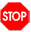05-arith-pretty
``{-# LANGUAGE GADTSyntax #-}``

# Module 05: The Arith language: pretty-printing

• Write your team names here:

• You may again choose whoever you want to start as the driver. Write your choice here:

## Arith syntax and semantics

The Arith language is represented by the following abstract syntax:

``````data Arith1 where
Lit1 :: Integer -> Arith1
Add  :: Arith1 -> Arith1 -> Arith1
Sub  :: Arith1 -> Arith1 -> Arith1
Mul  :: Arith1 -> Arith1 -> Arith1
deriving (Show)

arithExample :: Arith1
arithExample = Add (Mul (Lit1 4) (Lit1 5)) (Lit1 2)

arithExample2 :: Arith1
arithExample2 = Mul (Lit1 4) (Add (Lit1 5) (Lit1 2))``````

(We are using the name `Arith1` to avoid a name clash, since later we will use a more refined version called `Arith`.) The semantics of an Arith expression is an integer: `Lit1` values represent themselves, `Add` represents addition, `Sub` subtraction, and `Mul` multiplication. For example, `arithExample` evaluates to (4 × 5) + 2 = 22, and `arithExample2` evaluates to 4 × (5 + 2) = 28.

• Write an interpreter called `interpArith1` for `Arith1` expressions.

As concrete syntax for Arith, we use standard mathematical notation and standard conventions about operator precedence. For example, `"4*5+2"` is concrete syntax for `arithExample`, since by convention multiplication has higher precedence than addition. If we want concrete syntax to represent `arithExample2`, we have to use parentheses: `"4*(5+2)"`.

• Write a pretty-printer `prettyArith1` which turns Arith abstract syntax into valid concrete syntax. At this point, you should try to make your pretty-printer as simple as possible rather than try to produce the best output possible. (Hint: something like `"((4*5)+2)"` is just as valid concrete syntax as `"4*5+2"`, even though it has unnecessary parentheses.)

• How might you go about altering your pretty printer to omit needless parentheses? Write down some ideas here.## A better pretty-printer

If you have time and desire, you can try starting on this section. However, we will go over some things in class on Friday which will help a lot, so feel free to wait.

• ROTATE ROLES and write the name of the new driver here:
``````data Op where
Plus  :: Op
Minus :: Op
Times :: Op
deriving (Show, Eq)

data Arith where
Lit :: Integer -> Arith
Bin :: Op -> Arith -> Arith -> Arith
deriving (Show)

data Associativity where
L :: Associativity
R :: Associativity
deriving (Show, Eq)

type Precedence = Int

-- 4 * (5 + 2)
expr1 :: Arith
expr1 = Bin Times (Lit 4) (Bin Plus (Lit 5) (Lit 2))

-- 44 - 7 * (1 + 2) - 3
expr2 :: Arith
expr2 = Bin Minus (Lit 44) (Bin Minus (Bin Times (Lit 7) (Bin Plus (Lit 1) (Lit 2))) (Lit 3))``````
• First, write an interpreter `interpArith` for `Arith` expressions.

• Write functions `assoc :: Op -> Associativity` and `prec :: Op -> Precedence` to return the associativity and precedence of each operator. Addition, multiplication, and subtraction are all left-associative by convention. Addition and subtraction should have the same precedence level, with multiplication at a higher level (typically larger numbers represent higher precedence, that is, ``stickier glue’’).

• Now write a function `prettyPrec :: Precedence -> Associativity -> Arith -> String`. Given the precedence level of the parent operator and whether the current expression is a left or right child, it should print out a properly parenthesized version of the expression, with parentheses surrounding the entire expression only if they are needed. Remember that parentheses are needed when (and only when):

• The precedence of the parent operator is higher than the precedence of the current root operator, OR
• The precedence of the parent operator is equal to the precedence of the root operator, and the associativity of the root operator is the opposite of which side of the parent it is on.
• Write a function `prettyArith :: Arith -> String` which works by calling `prettyPrec` with appropriate starting arguments.

• Now go back and add an exponentiation operator to the language. Exponentiation is right-associative and has higher precedence than multiplication. Be sure to update both the interpreter and pretty-printer appropriately.